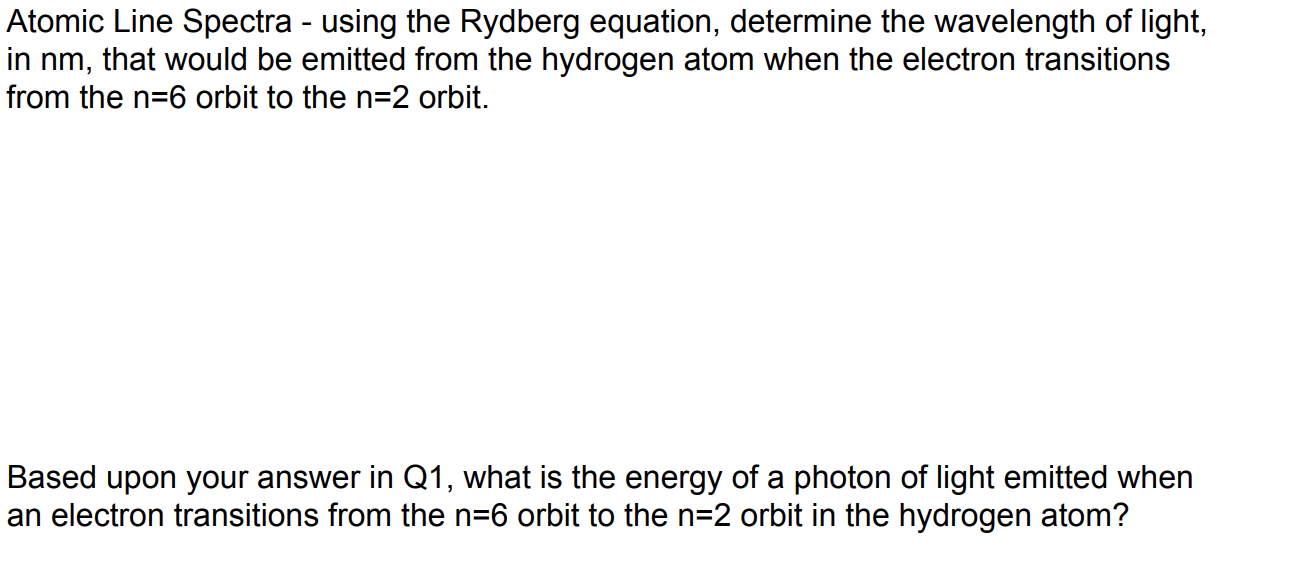# Atomic Line Spectra - using the Rydberg equation, determine the wavelength of light in nm, that would be emitted from the hydrogen atom when the electron transitions from the n-6 orbit to the n-2 orbit. Based upon your answer in Q1, what is thee energy of a photon of light emitted when an electron transitions from the n-6 orbit to the n-2 orbit in the hydrogen atom?

Questionhelp_outlineImage TranscriptioncloseAtomic Line Spectra - using the Rydberg equation, determine the wavelength of light in nm, that would be emitted from the hydrogen atom when the electron transitions from the n-6 orbit to the n-2 orbit. Based upon your answer in Q1, what is thee energy of a photon of light emitted when an electron transitions from the n-6 orbit to the n-2 orbit in the hydrogen atom? fullscreen